# 概率領域基本概念

## 離散型變數

### 概率分佈### 概率分佈函式F(x)

·對於離散型變數: $$F(x_i)=p(X<x_i)=\sum_{x_{k} < x_i} p_{k}$$

## 連續型變數

### 概率分佈函式F(x)

·對於離散型變數: $$F(x_i)=p(X<x_i)=\sum_{x_{k} < x_i} p_{k}$$ ·對於連續型變數，採用求積分的方式： $$F(x_i)=P(X < x_i)=\int_{-\infty}^{x_i} f(x) dx$$

（1）給出x落在某區間(a,b]內的概率： $$P(a<X \leq b)=F(b)-F(a)=\int_{a}^{b} f(x) d x$$ （2）根據F(x)的斜率,即概率密度函式f(x)判斷“區間概率”P(a<x≤b)（x落在(a,b]中的概率）。某區間(a,b]內，F(x)越傾斜，表示x落在該區間內的概率P(a<x≤b) 越大

### 概率密度函式f(x)：### 連續型變數的概率、概率分佈函式、概率密度函式之間的關係

x落在u附近的概率最大，而F(x)是概率的累加和，因此在u附近F(x)的遞增變化最快，即F(x)曲線在（u，F(u)）這一點的切線的斜率最大，這個斜率就等於f(u)。x落在a和b之間的概率為F(b)-F(a)（圖中的紅色小線段），而在概率密度曲線中則是f(x)與ab圍成的面積S。

## 常見概率分佈

### 離散分佈

• 離散均勻分佈： $X \sim U{1,2, \ldots, n}$

• 伯努利分佈： $X \sim B(p)=B(1, p)$

• 二項分佈： $X \sim B(n,p)$

### 連續分佈

• 均勻分佈： $X \sim U{a,b}$

• 指數分佈： $X \sim \operatorname{Exp}(\lambda)(\lambda>0)$• 正態分佈: $X \sim N(\mu,\sigma)$

# 未整理

## 置信區間詳解

### 答案1

1 點估計與區間估計• 大獎事先就固定好了，一定印在某一張刮刮卡上
• 買了刮刮卡之後，刮開就知道自己是否中獎

• 點估計：買一張，這就相當於你猜測這一張會中獎
• 區間估計：買一盒，這就相當於你猜測這一盒裡面會有某一張中獎

2 置信區間

2.1 上帝視角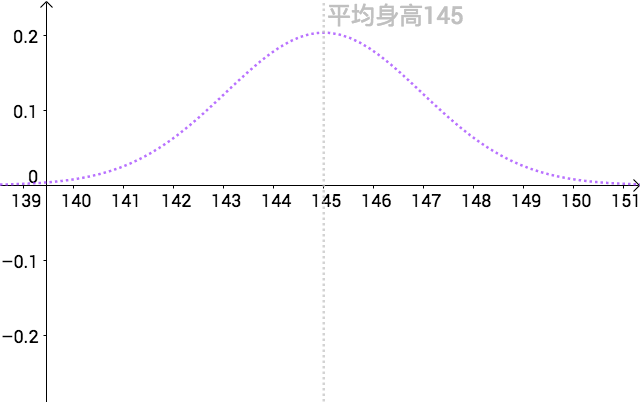2.2 點估計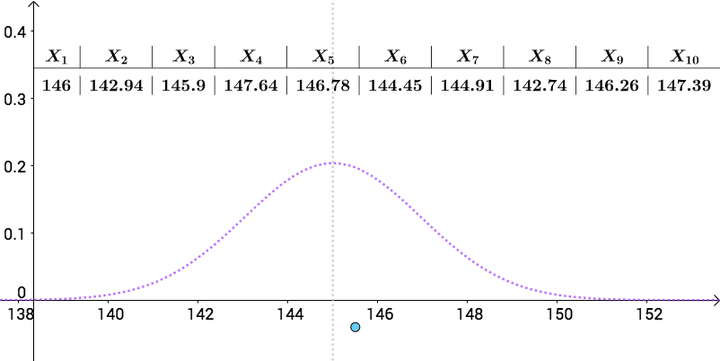就是對真實的的一次點估計。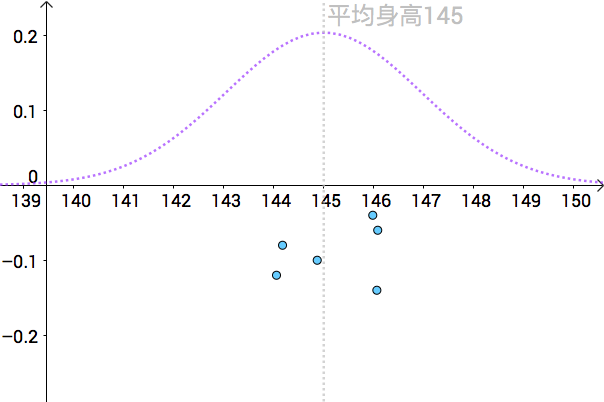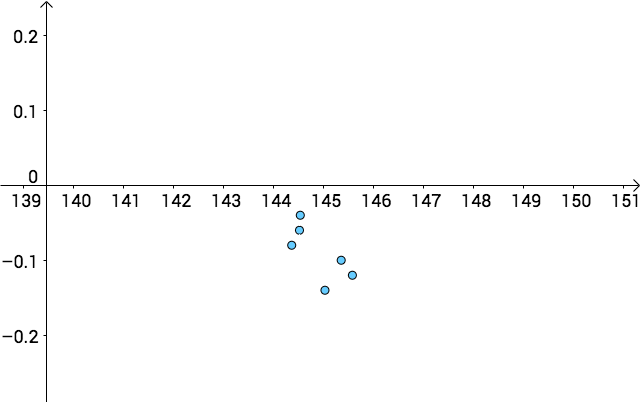2.3 置信區間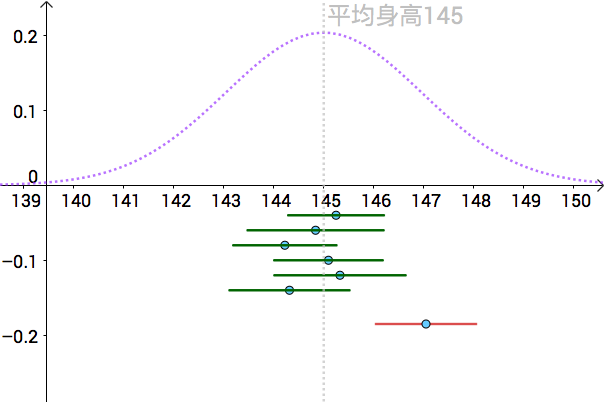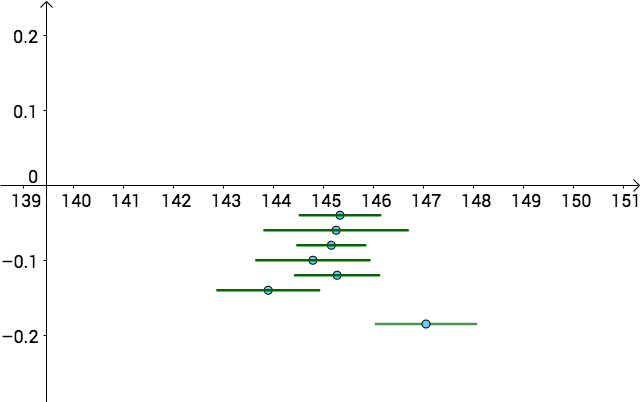• 點估計和區間估計，都不知道哪個點或者哪個區間更好
• 但是，按照置信區間構造出來的區間，如果我構造出100個這樣的區間，其中大約有95個會包含3置信區間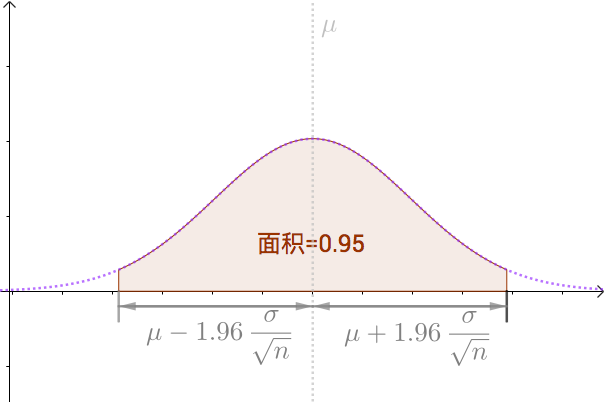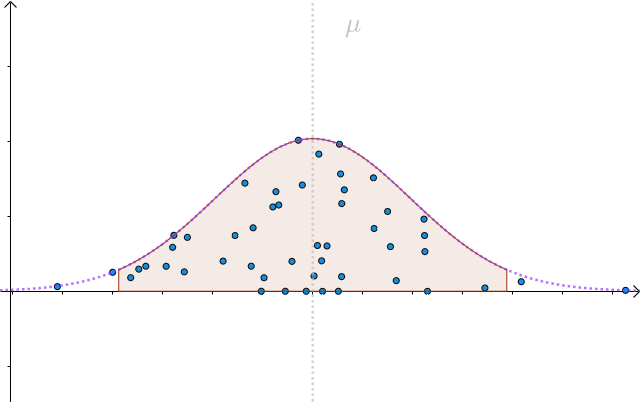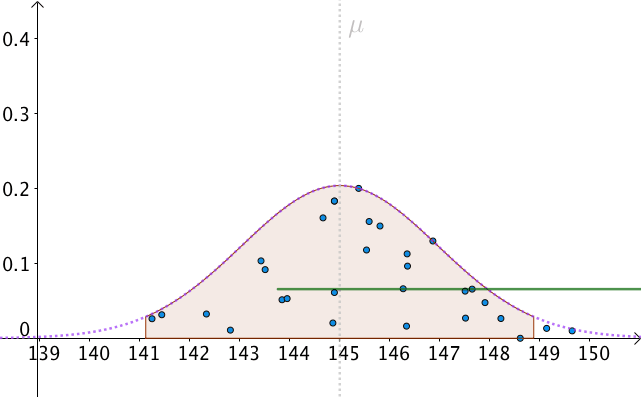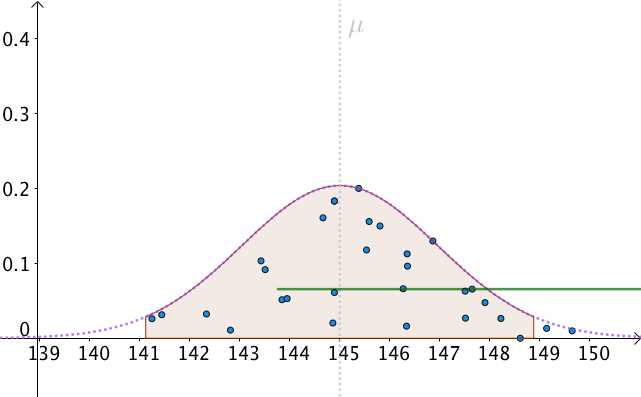4 總結

• 置信區間要求估計量是個常數
•也被稱為置信水平，是統計中的一個習慣，可以根據應用進行調整

[

236 人贊同了該回答

「其他文章」